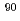For the application in the measurement of the high dose rate hot spots inside the Fukushima Daiichi Nuclear Power Station buildings, we propose a wavelength-resolved type one-dimensional radiation distribution sensing method using plastic scintillating fiber (PSF). The proposing method estimates the incident position of radiation to the PSF by the unfolding of the wavelength spectrum output from the fiber edge using the fact that the attenuation length of scintillating light depends on the wavelength. By measuring the response function in advance, which defined as the wavelength spectrum measured at the fiber edge by the spectrometer with every transmission distance, the spectrum which can obtain when measured a certain radiation distribution can be expressed as the convolution of the response function. This method can avoid the problem of chance coincidence effect and signal pile-up, which occurs in the radiation detector with pulse counting mode under high dose rate field because this method measures the integrated light intensity. Through basic experiment using the ultraviolet irradiation source andSr point source, basic properties of inverse estimation of irradiated position were confirmed, which showed that source position was reasonably estimated using the response function which obtained by the ultraviolet irradiation source in advance.